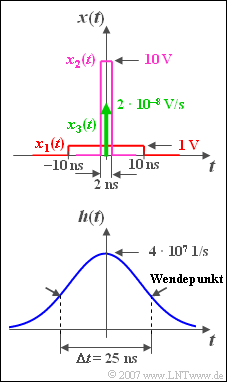# Exercise 3.9: Convolution of Rectangle and Gaussian PulseConvolution of rectangle  $x(t)$  and Gaussian pulse  $h(t)$

We consider a Gaussian low–pass with the equivalent bandwidth  $\Delta f = 40 \,\text{MHz}$:

$$H( f ) = {\rm{e}}^{{\rm{ - \pi }}( {f/\Delta f} )^2 } .$$

The corresponding impulse response is:

$$h( t ) = \Delta f \cdot {\rm{e}}^{{\rm{ - \pi }}( {\Delta f \hspace{0.05cm} \cdot \hspace{0.05cm} t} )^2 } .$$

From the sketch it can be seen that the  "equivalent time duration"   ⇒   $\Delta t = 1/\Delta f = 25\,\text{ns}$  of the impulse response  $h(t)$  can be read at the two inflection points of the Gaussian function.

Three different pulse-like signals are now applied to the input of the low-pass filter:

• a rectangular pulse  $x_1(t)$  with amplitude  $A_1 =1\,\text{V}$  and duration  $T_1 = 20\,\text{ns}$  (red curve),
• a rectangular pulse  $x_2(t)$  with amplitude  $A_2 =10\,\text{V}$  and duration  $T_2 = 2\,\text{ns}$  (violet curve),
• a Dirac delta  $x_3(t)$  with impulse weight  $2 \cdot 10^{–8}\text{ Vs}$  (green arrow).

Hints:

• To answer the questions, you can use the complementary Gaussian error integral, which is defined as follows:
$${\rm Q}( x ) = \frac{1}{ {\sqrt {2{\rm{\pi }}} }}\int_{\it x}^\infty {{\rm{e}}^{{{ - {\it u}}}^{\rm{2}} {\rm{/2}}} }\hspace{0.1cm}{\rm{d}}{\it u}.$$

This table gives some function values.

### Questions

1

Calculate the signal  $y_1(t) = x_1(t) \ast h(t)$.  What values result at times  $t = 0$  and  $t = 20\,\text{ns}$  with the approximation  $(2\pi )^{1/2} \approx 2.5$?

 $y_1(t=0)\ = \$  $\text{V}$ $y_1(t=20\,\text{ns})\ = \$  $\text{V}$

2

What are the signal values of the output signal  $y_2(t) = x_2(t) \ast h(t)$  at the considered time points?

 $y_2(t=0)\ = \$  $\text{V}$ $y_2(t=20 \,\text{ns})\ = \$  $\text{V}$

3

What is the value of the output signa  $y_3(t) = x_3(t) \ast h(t)$  at the considered time points?  Interpret the result.

 $y_3(t=0)\ = \$  $\text{V}$ $y_3(t=20\, \text{ns})\ = \$  $\text{V}$

### Solution

#### Solution

(1)  The convolution integral here is:

$$y_1( t ) = A_1 \cdot \Delta f \cdot \int_{t - T_1 /2}^{t + T_1 /2} {{\rm{e}}^{{\rm{ - \pi }}( {\Delta f \hspace{0.05cm}\cdot \hspace{0.05cm} \tau } )^2 } }\hspace{0.1cm} {\rm{d}}\tau = \frac{A_1 }{\sqrt{2\pi }} \cdot\int_{u_1 }^{u_2 } {{\rm{e}}^{ - u^2 /2}\hspace{0.1cm} {\rm{d}}u.}$$
• Here the substitution  $u = \sqrt {2{\rm{\pi }}} \cdot \Delta f \cdot \tau$  was used.  The integration limits are at:
$$u_1 = \sqrt {2{\rm{\pi }}} \cdot \Delta f \cdot \big( {t - T_1 /2} \big),\hspace{0.5cm}u_2 = \sqrt {2{\rm{\pi }}} \cdot \Delta f \cdot \big( {t + T_1 /2} \big).$$
• Using the complementary Gaussian error integral, it is also possible to write for this:
$$y_1 (t) = A_1 \cdot \big[ {{\rm Q} ( {u_1 } ) - {\rm Q}( {u_2 } )} \big].$$
• For time  $t = 0$  one obtains with  $(2\pi )^{1/2} \approx 2.5$:
$$u_2 = \sqrt {2{\rm{\pi }}} \cdot \Delta f \cdot \frac{ {T_1 }}{2} \approx 2.5 \cdot 4 \cdot 10^{7} \;{\rm{1/s}} \cdot 10^{-8} \;{\rm{s}} = 1.$$
• With  $u_1 = -u_2 = -1$ , it follows for the two signal values we are looking for:
$$y_1 ( {t = 0} ) \approx A_1 \cdot \big[ {{\rm Q}( { - 1} ) - {\rm Q}(+ 1 )} \big] = 1\;{\rm{V}} \cdot \big[ {{\rm{0}}{\rm{.841 - 0}}{\rm{.159}}} \big] \hspace{0.15 cm}\underline{= 0.682\;{\rm{V}}}{\rm{,}}$$
$$y_1 ( {t = 20\;{\rm{ns}}} ) \approx A_1 \cdot \big[ {{\rm Q}( 1 ) - {\rm Q}( 3 )} \big] = 1\;{\rm{V}} \cdot \big[ {{\rm{0}}{\rm{.159 - 0}}{\rm{.001}}} \big] \hspace{0.15 cm}\underline{= 0.158\;{\rm{V}}}{\rm{.}}$$

(2)  Analogous to the first sample solution, one obtains  $x_2(t)$ for the narrower input pulse:

$$y_2 ( {t = 0} ) \approx A_2 \cdot \big[ {{\rm Q}( { - 0.1} ) - {\rm Q}( {0.1} )} \big] = 10\;{\rm{V}} \cdot \big[ {{\rm{0}}{\rm{.540 - 0}}{\rm{.460}}} \big] \hspace{0.15 cm}\underline{= 0.80\;{\rm{V}}}{\rm{,}}$$
$$y_2 ( {t = 20\,{\rm ns}} ) \approx A_2 \cdot \big[ {{\rm Q}( {1.9} ) - {\rm Q}( {2.1} )} \big] = 10\;{\rm{V}} \cdot \big[ {{\rm{0}}{\rm{.029 - 0}}{\rm{.018}}} \big] \hspace{0.15 cm}\underline{= 0.11\;{\rm{V}}}{\rm{.}}$$

(3)  With the Dirac delta  $x_3(t)$ , the output signal  $y_3(t)$  is equal to the impulse response  $h(t)$, weighted by the weight of the Dirac function:

$$y_3 (t) = 2 \cdot 10^{ - 8} \,{\rm{Vs}} \cdot 4 \cdot 10^7 \;{\rm{1/s}} \cdot {\rm{e}}^{ - {\rm{\pi }}( {\Delta f \cdot t})^2 }.$$
• At time  $t = 0$ , one also obtains here with a good approximation  $y_3( t=0)\hspace{0.15 cm}\underline{ =0.8\, {\rm V}}$.
• After  $20\, \rm ns$ , the output pulse is smaller by a factor of  ${\rm e}^{–0.64π} \hspace{0.15 cm}\underline{\approx 0.136}$  and one obtains  $y_3( t = 20 \,\text{ns}) ≈ 0.11 \,\text{V}$.

One can see from comparing the results from  (2)  and   (3), that  $y_3(t) \approx y_2(t)$  gilt.

• The reason for this is that the Dirac delta is a good approximation for a rectangular input impulse of the same area if the rectangular duration  $T$  is significantly smaller than the equivalent impulse duration  $\Delta t$  of the impulse response.
• This means for our example:   If the duration  $T$  of the rectangular input impulse  $x(t)$  is clearly smaller than the  "equivalent pulse duration"  $\Delta t$  of the Gaussian impulse response  $h(t)$, then  $y(t)$  is also almost Gaussian.  But:   Gaussian (once) folded with non–Gaussian never results in (exactly) Gaussian!